Real Numbers: Property CHART MathBitsNotebook.com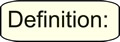A real number is a value that represents a quantity along a continuous number line. Real numbers can be ordered. The symbol for the set of real numbers is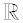, which is the letter R in the typeface "blackboard bold".
 The real numbers include: counting (natural) numbers (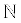){1, 2, 3, ... }, whole numbers {0, 1, 2, 3, ... }, integers (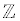){... , -3, -2, -1, 0, 1, 2, 3, ...}, rational numbers (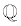) (such as -½, 6.25,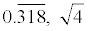) and irrational numbers (such as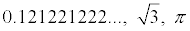).
FYI: The series of three dots ( ... ), seen within the sets above, is called an ellipsis.
As used in mathematics, the ellipsis means "and so forth".

The properties of the Real Number System will prove useful when working with equations, functions and formulas in Algebra, as they allow for the creation of equivalent expressions which will often aid in solving problems. In addition, they can be used to help explain or justify solutions.

 Property (a, b and c are real numbers, variables or algebraic expressions) Examples Verbal hints 1. Distributive Property a • (b + c) = a • b + a • c 3 • (4 + 5) = 3 • 4 + 3 • 5 "multiplication distributes across addition" 2. Commutative Property of Addition a + b = b + a 3 + 4 = 4 + 3 "commute = to get up and move to a new location : switch places" 3. Commutative Property of Multiplication a • b = b • a 3 • 4 = 4 • 3 "commute = to get up and move to a new location: switch places" 4. Associative Property of Addition a + (b + c) = (a + b) + c 3 + (4 + 5) = (3 + 4) + 5 "regroup - elements do not physically move, they simply group with a new friend." 5. Associative Property of Multiplication a • (b • c) = (a • b) • c 3 • (4 • 5) = (3 • 4) • 5 "regroup - elements do not physically move, they simply group with a new friend." 6. Additive Identity Property a + 0 = a 4 + 0 = 4 "the value that returns the input unchanged" 7. Multiplicative Identity Property a • 1 = a 4 • 1 = 4 "the value that returns the input unchanged" 8. Additive Inverse Property a + (-a) = 0 4 + (-4) = 0 "the value that brings you back to the identity element under addition" 9. Multiplicative Inverse Property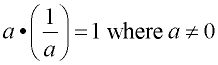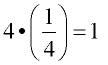"the value that brings you back to the identity element under multiplication" 10. Zero Property of Multiplication a • 0 = 0 4 • 0 = 0 "zero times any value is 0" 11. Closure Property of Addition a + b is a real number 10 + 5 = 15 (a real number) "the sum of any two real numbers is another real number" 12. Closure Property of Multiplication a • b is a real number 10 • 5 = 50 (a real number) "the product of any two real numbers is another real number" 13. Addition Property of Equality If a = b, then a + c = b + c. If x = 10, then x + 3 = 10 + 3 "adding the same value to both sides of an equation will not change the truth value of the equation." 14. Subtraction Property of Equality If a = b, then a - c = b - c. If x = 10, then x - 3 = 10 - 3 "subtracting the same value from both sides of an equation will not change the truth value of the equation." 15. Multiplication Property of Equality If a = b, then a • c = b • c. If x = 10, then x • 3 = 10 • 3 "multiplying both sides of an equation by the same value will not change the truth value of the equation." 16. Division Property of Equality If a = b, then a / c = b / c, assuming c ≠ 0. If x = 10, then x / 3 = 10 / 3 "dividing both sides of an equation by the same non-zero value will not change truth value of the equation." 17. Substitution Property If a = b, then a may be substituted for b, or conversely. If x = 5, and x + y = z, then 5 + y = z. "a value may be substituted for its equal." 18. Reflexive (or Identity) Property of Equality a = a 12 = 12 "a real number is always equal to itself" 19. Symmetric Property of Equality If a = b, then b = a.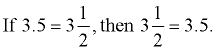"quantities that are equal can be read forward or backward" 20. Transitive Property of Equality If a = b and b = c, then a = c. If 2a = 10 and 10 = 4b, then 2a = 4b. "if two numbers are equal to the same number, then the two numbers are equal to each other" 21. Law of Trichotomy Exactly ONE of the following holds: a < b, a = b, a > b If 8 > 6, then 8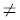6 and 8 is not < 6. "for two real numbers a and b, a is either equal to b, greater than b, or less than b." (common sense)

FYI: Regarding the term "natural numbers", there is no universal agreement about whether to include zero in this set. Most mathematicians hold with the older traditional and define natural numbers to be the counting numbers [positive integers {1, 2, 3, ...}]. Computer scientists, set theorists, logicians and other mathematicians define natural numbers to be the whole numbers [non-negative integers {0, 1, 2, ...}]. This site will use the term "natural numbers" to refer to the counting numbers {1, 2, 3, ...}.